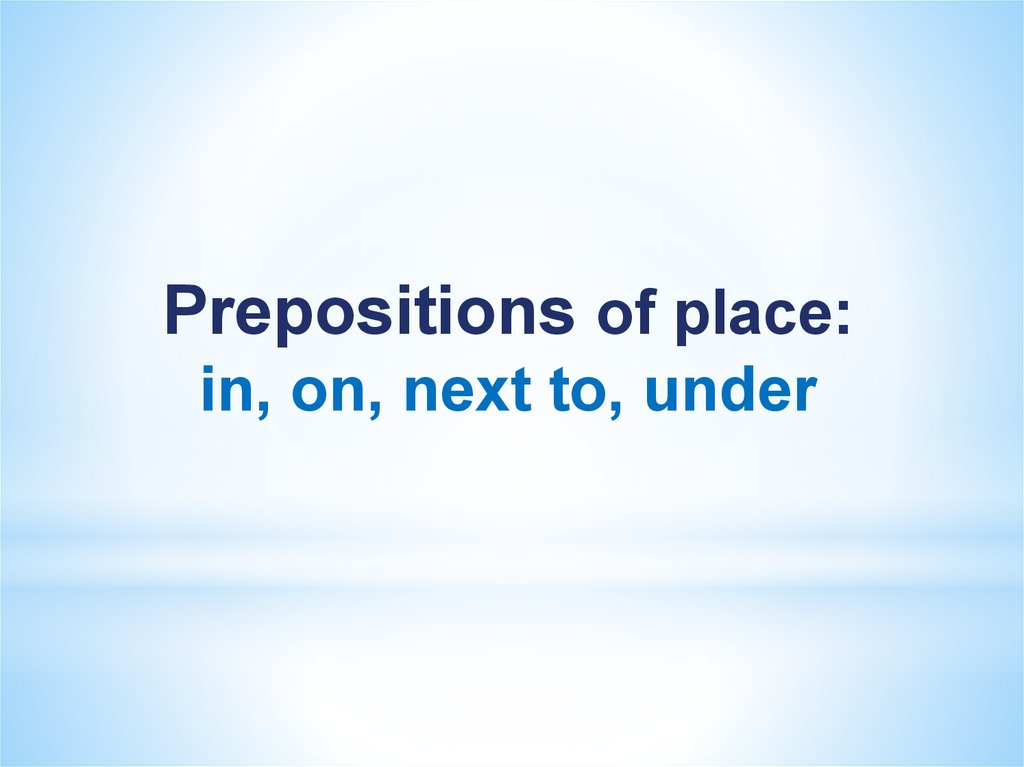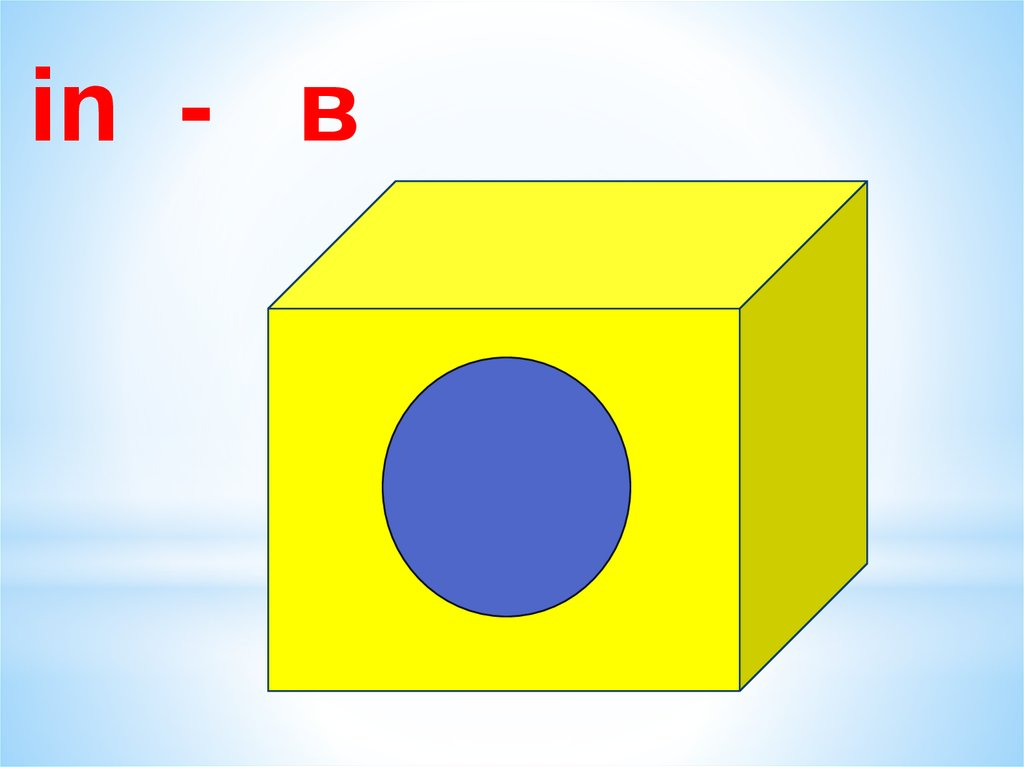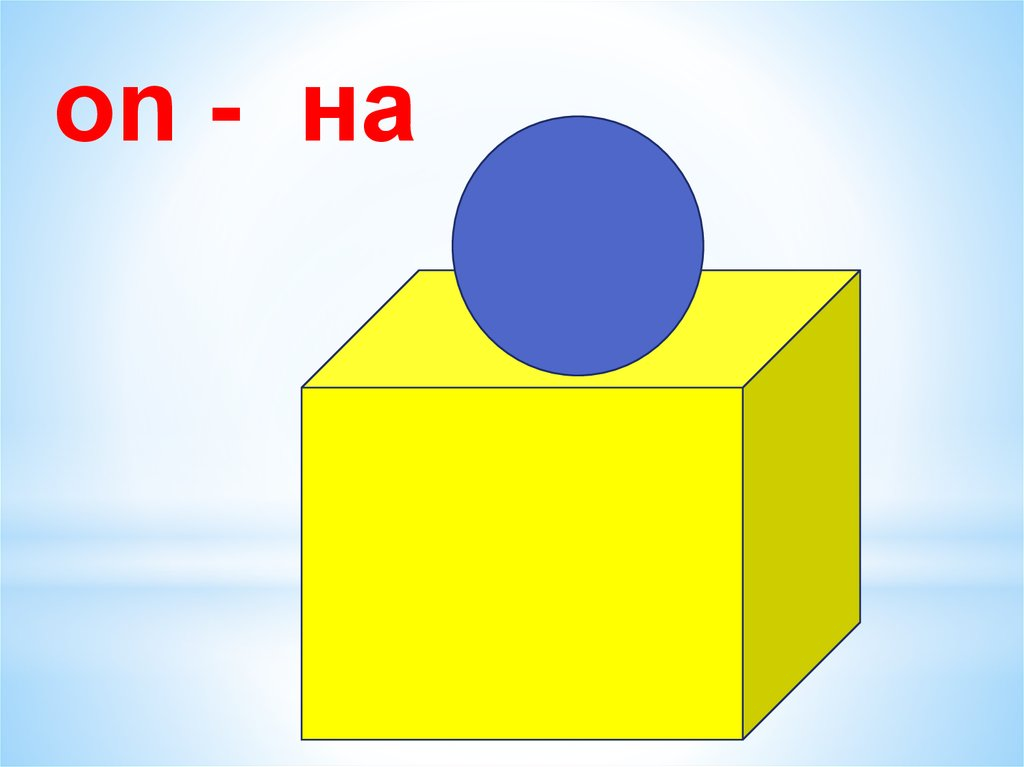# Prepositions of place: in, on, next to, under

## 1.

Prepositions of place:
in, on, next to, under

in - в

on - на

## 4.

next to - рядом с

under - под

## 6.

Where is the phone?
The phone is on the table.

## 7.

Where are the keys?
The keys are under the chair.

## 8.

Where is the pencil box?
The pencil box is on the floor.

## 9.

Where is the camera?
The camera is next to the phone.

## 10.

Where is the wallet?
The wallet is under the chair.

## 11.

Where are the books?
The books are in the bag.

## 12.

Where is the pen?
The pen is on the chair.

## 13.

Where is the bag?
The bag is under the table.

## 14.

Where is the table?
The table is next to the chair.

## 15.

Where is the pencil?
The pencil is on the chair.

## 16.

Correct the preposition in the sentences.
The phone is in the table.

## 17.

Correct the preposition in the sentences.
The keys are on the chair.

## 18.

Correct the preposition in the sentences.
The camera is under the phone.

## 19.

Correct the preposition in the sentences.
The wallet is on the chair.

## 20.

Correct the preposition in the sentences.
The pencil box is in the bag.